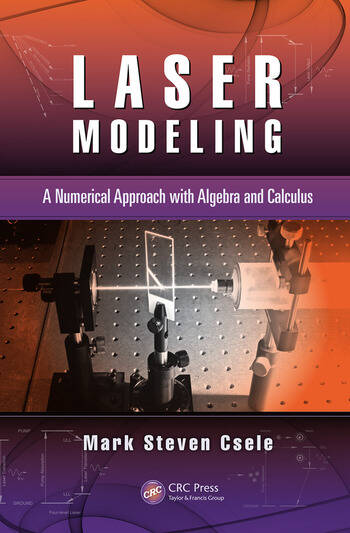Laser Modeling: A Numerical Approach with Algebra and Calculus

1st Edition

Mark Steven Csele

CRC Press
Published March 29, 2017
Reference - 274 Pages - 157 B/W Illustrations
ISBN 9781138071995 - CAT# K33762

For Instructors Request Inspection Copy

was \$86.95

USD\$69.56

SAVE ~\$17.39

FREE Standard Shipping!

Preview

Summary

Offering a fresh take on laser engineering, Laser Modeling: A Numerical Approach with Algebra and Calculus presents algebraic models and traditional calculus-based methods in tandem to make concepts easier to digest and apply in the real world. Each technique is introduced alongside a practical, solved example based on a commercial laser. Assuming some knowledge of the nature of light, emission of radiation, and basic atomic physics, the text:

• Explains how to formulate an accurate gain threshold equation as well as determine small-signal gain
• Discusses gain saturation and introduces a novel pass-by-pass model for rapid implementation of "what if?" scenarios
• Outlines the calculus-based Rigrod approach in a simplified manner to aid in comprehension
• Considers thermal effects on solid-state lasers and other lasers with new and efficient quasi-three-level materials
• Demonstrates how the convolution method is used to predict the effect of temperature drift on a DPSS system
• Describes the technique and technology of Q-switching and provides a simple model for predicting output power
• Addresses non-linear optics and supplies a simple model for calculating optimal crystal length
• Examines common laser systems, answering basic design questions and summarizing parameters
• Includes downloadable Microsoft® Excel spreadsheets, allowing models to be customized for specific lasers

Don’t let the mathematical rigor of solutions get in the way of understanding the concepts. Laser Modeling: A Numerical Approach with Algebra and Calculus covers laser theory in an accessible way that can be applied immediately, and numerically, to real laser systems.

Instructors

We provide complimentary e-inspection copies of primary textbooks to instructors considering our books for course adoption.